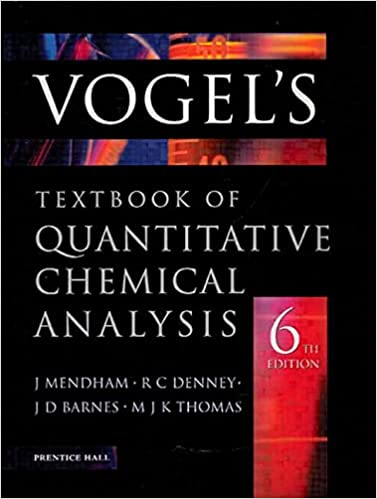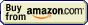# Acid-base titration## Acid-base titration » Standard substances

Remember to use analytical reagents (AR grade) for standards.

Borax Na2B4O7·10H2O - before weighting keep in a hygrostat over NaBr·2H2O. Equivalent weight is one-half the formula weight (381.3721 g).

Sodium carbonate Na2CO3 - heat for 1 hour at 270-300°C. Equivalent weight is one-half the formula weight (105.9884 g).

Potassium hydrogen phthalate (KHP, KHC8H4O4) - dry for 2 hours at 120° C. Equivalent weight is 204.2212 g.

Potassium hydrogen bis(iodate) KH(IO3)2 - dry at 110°C. Equivalent weight is 389.9116 g.